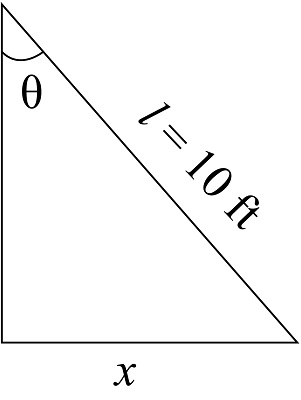# A ladder 10 ft long rests against a vertical wall. Let \theta be the angle between the top of the...

## Question:

A ladder {eq}10 ft {/eq} long rests against a vertical wall. Let {eq}\theta {/eq} be the angle between the top of the ladder and the wall and let {eq}x {/eq} be the distance from the bottom of the ladder to the wall.

If the bottom of the ladder slides away from the wall, how fast does {eq}x {/eq} change with respect to {eq}\theta {/eq} when {eq}\theta = \pi/3 {/eq}

## Differentiation:

Differentiation is used to find the rate of change of some physical quantity. It basically tells about the slope . For example, the rate of change of velocity is referred to as acceleration.

The figure below shows the schematic diagram of the wall.The angle {eq}\theta {/eq} is:

{eq}\begin{align*} \sin \theta &= \dfrac{x}{l}\\ x &= l\sin \theta \end{align*} {/eq}

Differentiate the above equation.

{eq}\dfrac{{dx}}{{d\theta }} = l\cos \theta {/eq}

Substitute {eq}l = 10{\rm{ ft}} {/eq} and {eq}\theta = \dfrac{\pi }{3} {/eq} in the above equation.

{eq}\begin{align*} \dfrac{{dx}}{{d\theta }} &= 10\cos \left( {\dfrac{\pi }{3}} \right)\\ &= 5 \end{align*} {/eq}

Thus the rate of change of x with respect to {eq}\theta {/eq} is 5.